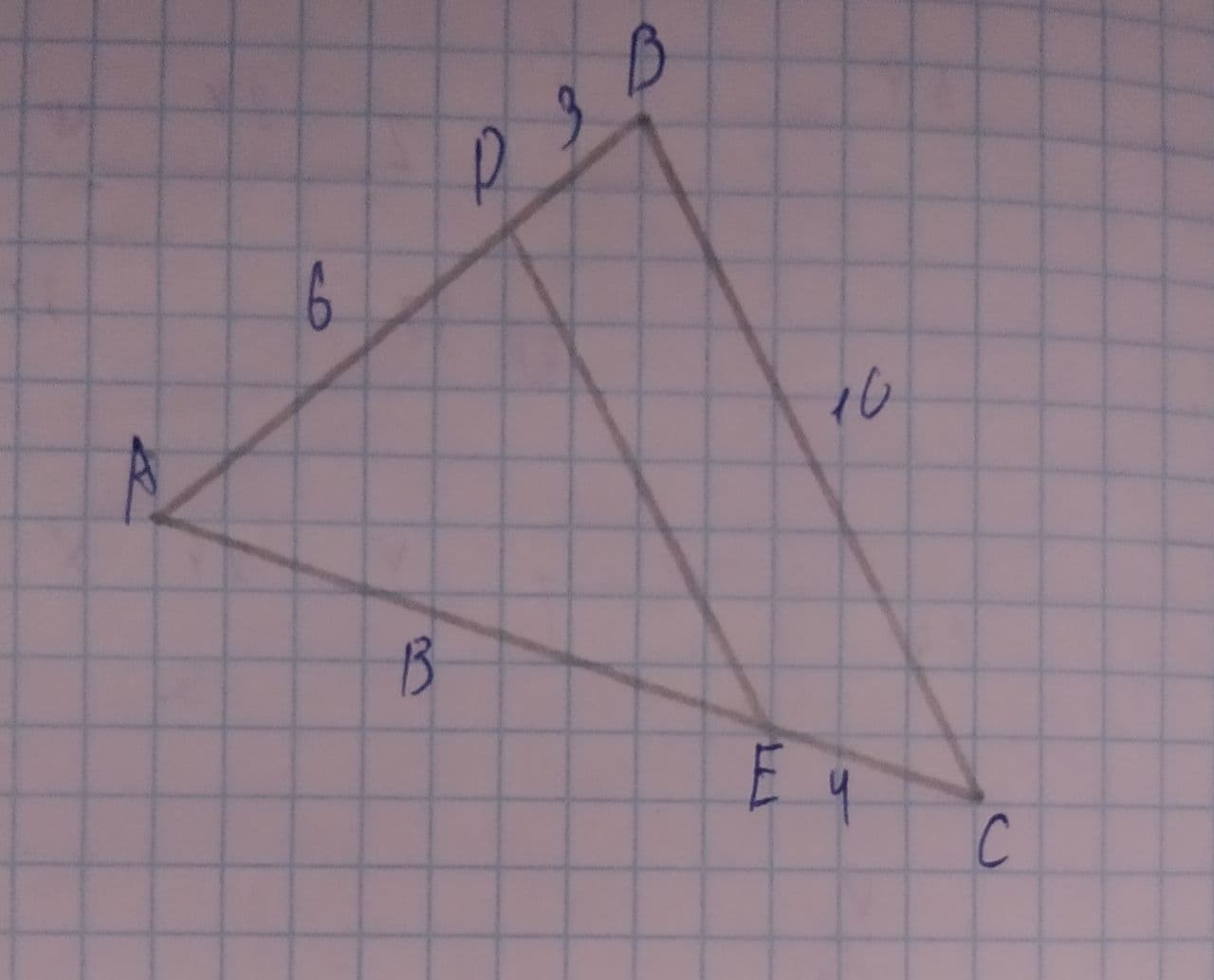# Are the two triangles similar? If so, by what similarity shortcut?coexpennan 2021-08-06 Answered
Are the two triangles similar? If so, by what similarity shortcut?SSS
SAS
AA
Not Similar
You can still ask an expert for help

• Questions are typically answered in as fast as 30 minutes

Solve your problem for the price of one coffee

• Math expert for every subject
• Pay only if we can solve itDelorenzoz

Since $\mathrm{\angle }EAC=\mathrm{\angle }CAB$ (same angle)
and $\mathrm{\angle }ADE=\mathrm{\angle }ABC$ (DE and BC are parallel)
$⇒$ Hence the $\mathrm{△}$'s are similar by AA